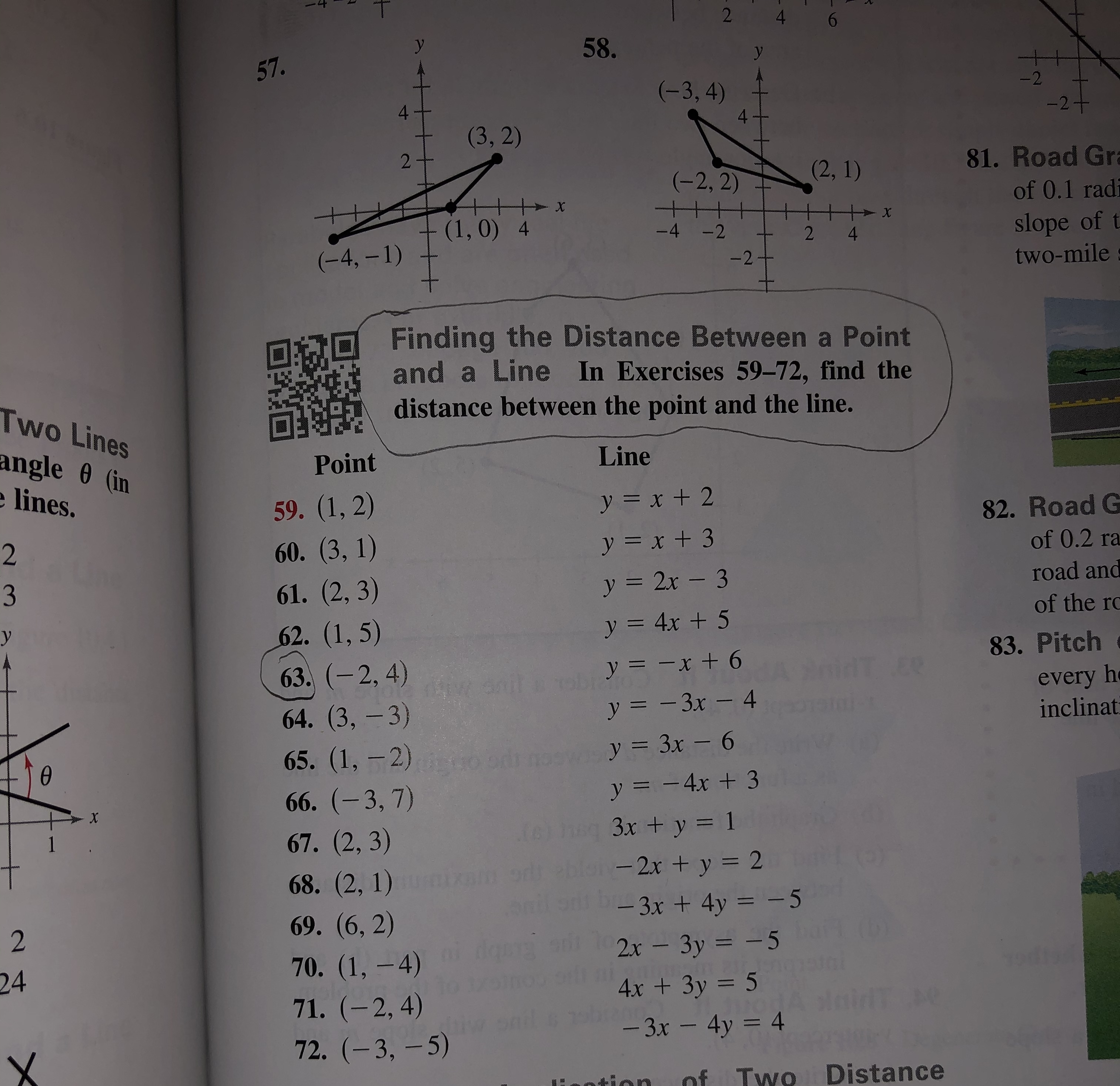# 246.58.57.-2(-3, 4)4-24.(3, 2)281. Road Gra(2, 1)(-2, 2)of 0.1 radiх(1, 0) 4slope of ttwo-mile-4 -24(-4, – 1)-2Finding the Distance Between a Pointand a Line In Exercises 59-72, find thedistance between the point and the line.Two Linesangle 0 (ine lines.LinePoint59. (1, 2)60. (3, 1)61. (2, 3)62. (1, 5)y = x + 282. Road Gof 0.2 ray = x + 3road and3y = 2x – 3of the rcy = 4x + 583. Pitch63. (-2, 4)y = -x + 6LEeevery heinclinaty = - 3x – 464. (3,-3)y = 3x – 665. (1, -2)d66. (-3, 7)67.(2, 3)%3Dy = - 4x + 3eg 3x + y = 1blo-2x + y = 2a 3x + 4y = -5%3D168. (2, 1)%3D69. (6, 2)2x - 3y = - 570. (1, -4)244x + 3y = 5%3D71. (-2, 4)- 3x - 4y = 4%3D72. (-3, -5)of Two Distanceotion2.

Question
20 views

How do you answer number 63 with the given instructions above it?help_outlineImage Transcriptionclose2 4 6. 58. 57. -2 (-3, 4) 4 -2 4. (3, 2) 2 81. Road Gra (2, 1) (-2, 2) of 0.1 radi х (1, 0) 4 slope of t two-mile -4 -2 4 (-4, – 1) -2 Finding the Distance Between a Point and a Line In Exercises 59-72, find the distance between the point and the line. Two Lines angle 0 (in e lines. Line Point 59. (1, 2) 60. (3, 1) 61. (2, 3) 62. (1, 5) y = x + 2 82. Road G of 0.2 ra y = x + 3 road and 3 y = 2x – 3 of the rc y = 4x + 5 83. Pitch 63. (-2, 4) y = -x + 6 LEe every he inclinat y = - 3x – 4 64. (3,-3) y = 3x – 6 65. (1, -2)d 66. (-3, 7) 67.(2, 3) %3D y = - 4x + 3 eg 3x + y = 1 blo-2x + y = 2 a 3x + 4y = -5 %3D 1 68. (2, 1) %3D 69. (6, 2) 2x - 3y = - 5 70. (1, -4) 24 4x + 3y = 5 %3D 71. (-2, 4) - 3x - 4y = 4 %3D 72. (-3, -5) of Two Distance otion 2. fullscreen
check_circle

star
star
star
star
star
1 Rating
Step 1

The given point and the line is,

Step 2

The formula for obtaining the distance, d betwe...

### Want to see the full answer?

See Solution

#### Want to see this answer and more?

Solutions are written by subject experts who are available 24/7. Questions are typically answered within 1 hour.*

See Solution
*Response times may vary by subject and question.
Tagged in

### Equations and In-equations